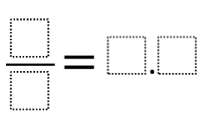Home > Grade 4 > Fraction and Decimal

# Fraction and Decimal

Directions: Using the digits 0 through 9, at most one each time, create an an equivalent fraction and decimal number.### Hint

How can you rewrite the fraction in decimal form?

There are many possibilities, here are some: 1/2 = 0.5
2/4 = 0.5
3/6 = 0.5
4/8 = 0.5

Source: Giselle Garcia

## Multiplying Two-Digit Numbers – Closest to 7,000

Directions: Directions: Using the digits 1 to 9 at most one time each, fill in …

1.We had a variety of answers on this one, but one I did not expect was 7/ 3 4/8 = 2.0 Although I generally steer clear of complex fractions I thought this was a creative way to solve this “puzzle.”

2.I figured out all the answers in less than a second

3.1/5 = 0.2
2/5 = 0.4
3/5 = 0.6
4/5 = 0.8
3/2 = 1.5

If you allow two-digit numbers, it gets more fun:
48/30 = 1.6
34/20 = 1.7
… and I’m sure, many more.

4.A student and I found 19 different answers, the halves from above, the fifths from above, as well as some impropers like
7/5 =1.4
6/5 = 1.2
6/2 = 3.0
7/2 = 3.5
8/2 = 4.0
9/2 = 4.5
8/4 = 2.0
This was a fun one!

5.58/100 = 0.58

•Good try, Ale, but remember that you can only use the digits at most one each time.

6.Isabella Mendoza

Isabella Mendoza

4/8 =0.5

7.right away i knew one.
1/4 0.25

8.3/4 = 0.75